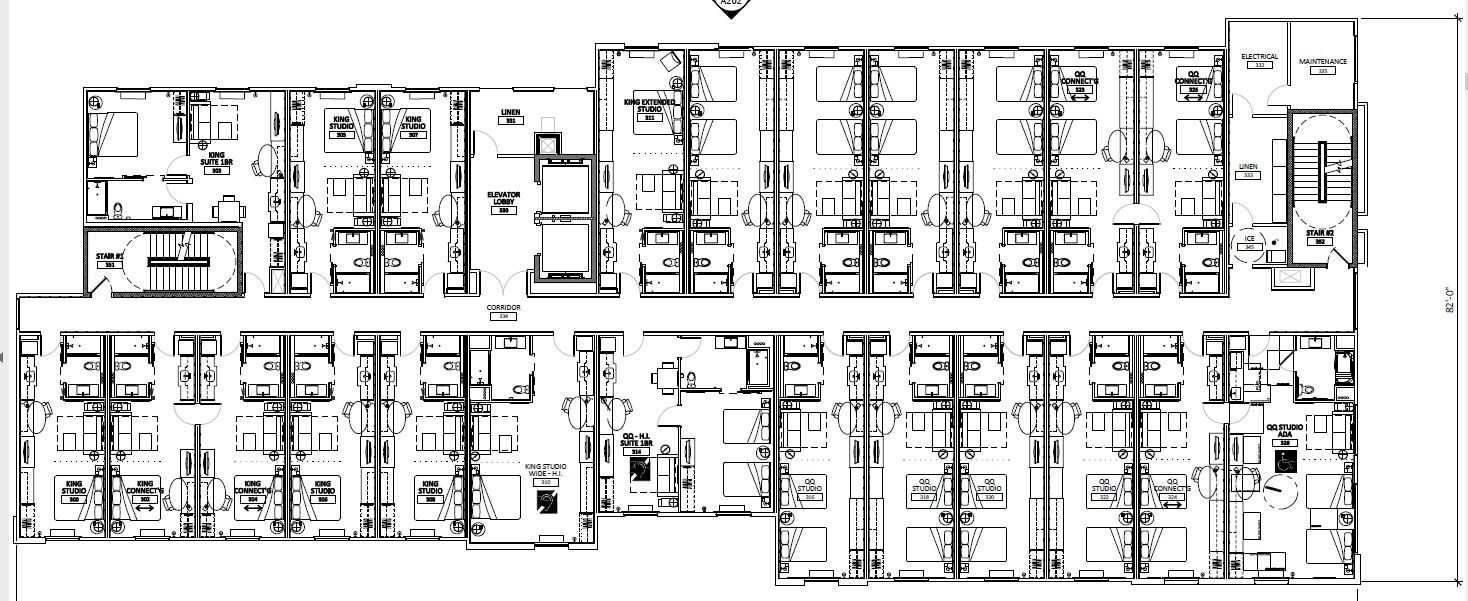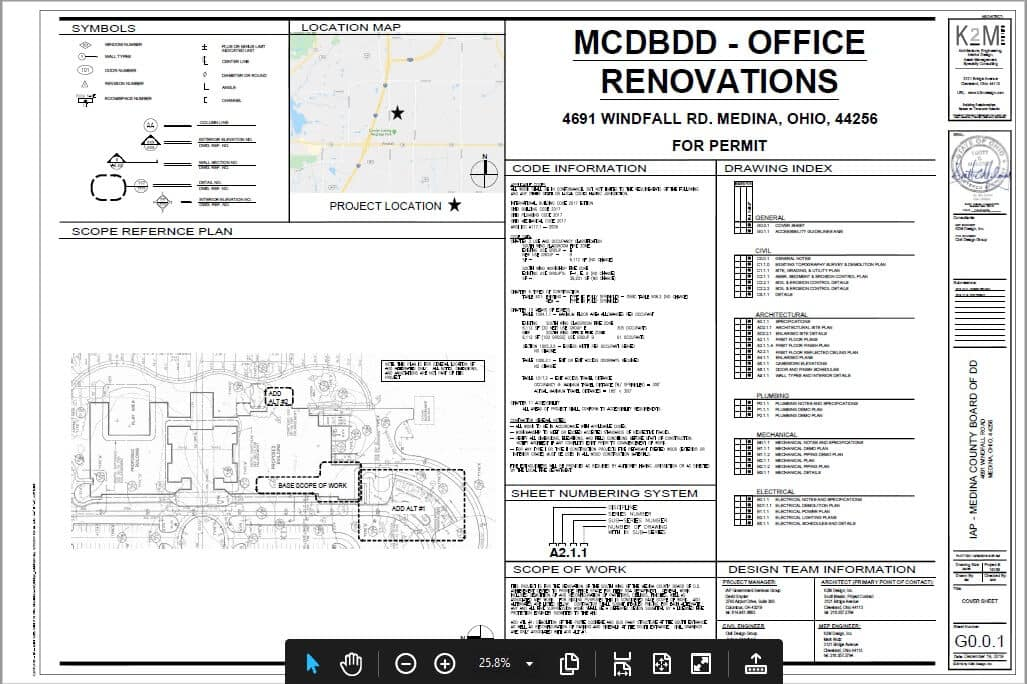# 8 Types of Cost Estimates in Project Management

There are different types of cost estimates that are involved in project management. In the following blog, we will be discussing them and will also look at the meaning of estimate and its purpose.

## What is An Estimate?

An estimate is a calculation done for the quantities of a different item of work along with their expenses that are involved in the project work. The sum cost of all the quantity of work by a construction cost estimator will give an idea about the total construction cost or estimated cost of project work. Note that the calculated cost of work will be a close approximation of its actual cost. A correct and accurate estimate is necessary for all the projects to make it cost-effective.

## Purpose of Estimating

The primary purpose of the estimation by a construction estimator is to provide a reasonably accurate idea of the cost. The accurate estimation cost of the project is essential to give the owner a reasonably accurate idea of the cost that will help him to decide whether he has enough finance for the work or need to arrange some more money.

## Types of Cost Estimates Used in Construction

Now, let’s have a look at the different types of project cost estimation that is used in construction:

## 1-Preliminary Cost Estimate

The preliminary cost estimating is also called as the approximate cost estimate, abstract cost estimate, or budget estimate. Generally, it is prepared in the preliminary phase of the project planning to get an idea about the approximate cost of the project. With the help of this estimate, the project authority will know the financial position and policy needed for the administration section.The preliminary cost of the project is evaluated by the cost of similar type projects practically. This estimate, the cost of an important item of work is calculated individually to understand the necessity and utility of every item of work. In this estimate, an important item of work includes the cost of lands, electrification, cost of roads, water supply costs, cost of each building, etc.

## 2-Cube Rate Cost Estimate

The cubical content of the building is calculated by multiplying the plinth area with the height of the building. The height of the building will be from floor level to the top of the roof level. This method is best for high rise buildings. The cube rate cost estimate method is accurate in comparison to the plinth area estimate. The cubical rate of construction is obtained by dividing total construction costs by its cubical content.• The cost for the proposed project is calculated by multiplying the cubic rate of similar types of buildings located in a particular location with a cubic content of future work.
• Let’s suppose, the cubic rate in an area is USD 330.33 / m3 and the area of the proposed construction is 1000m3
• Approximate cost of construction = 330.33 x 1,000 = USD 330,330.oo

## 3-Plinth Area Cost Estimate

Next, the plinth area estimate is involved in the types of cost estimates. This cost estimate in project management is prepared by considering the cost per plinth area of a similar type of construction present in the locality. It is an area that is covered by external dimensions of building at the floor level and the plinth area rate of the building that is the cost of similar buildings with specifications located in that particular locality.

• The plinth area estimate for a building is prepared by multiplying the plinth area of building with the plinth area rate.
• For instance, if the plinth area is 100 sq.m and plinth area rate of a building in the same locality is 2000 per sq.m then,
• The cost for the building by the plinth area estimate will be 100 X 2000 = 200000.
• The open areas, courtyards, etc. should not be included in the plinth area.  In the case of a multi-storied construction, the cost of each floor should be calculated separately.

## 4-Detailed Cost Estimate

A detailed estimate will be prepared after the approval of a preliminary estimate by the competent administrative authority. In this estimate, every item of work will be measured accurately and the cost will be calculated separately. This construction cost estimating is one of the most accurate estimates as compared to the other method of estimation.

The cost of any item of work is calculated from the current market rates along with the total estimated cost. For the miscellaneous expenditure, an amount of 3 to 5 % of the estimated cost will be added to the total estimated cost. A detailed estimate is an important estimate required to get the final financial sanction from a competent authority.

The detailed estimate will include the following details and documents. A detailed report will have the following things:

• General Specifications
• Detailed Specifications
• Drawings/plans: elevation, sectional views, layout plans, detailed drawings, etc.
• Designs and calculations: In the case of buildings design of foundations, beams, slab, etc.

## 5-Approximate Quantity Method Cost Estimate

In this estimate, the total length of the wall of the whole construction is calculated and this length will be multiplied by the rate per running meter that will give you the cost of the building, foundation, and superstructure rate per running. Furthermore, the meter is calculated separately.

For the cost estimation of the foundation rate per running, the meter is calculated by considering the quantities like excavation cost and brickwork cost up to plinth. The superstructure quantities will include the brickwork for the wall, floor finishing, woodworks, and more are considered for the deciding rate per running meter.

## 6-Revised Cost Estimate

The revised estimate is prepared when the original cost of the project is exceeded by 5% or more. It is a detailed estimate but an improper estimating or underestimated cost of the project that needs to be revised.

The reason behind the preparation of the revised estimate might increase in the cost of materials, cost of transportation, and much more. The major reason behind the preparation of this estimate needs to be mentioned on the last page of the revised estimate. For this, you need to get the construction estimating services by experts.

## 7-Annual Repair Cost Estimate

The annual repair cost estimate is called the annual maintenance estimate that is prepared to get an idea about the costs of maintenance of the building that will keep the structure in a safe condition. This will include the cost of whitewashing, painting, minor repairs, and more are considered while preparing the annual repair estimate for a building.## 8-Supplementary Cost Estimate

The supplementary cost estimate is a supplement to your detailed estimate and it should be prepared freshly when there is a need for additional works during the progress of original work. Furthermore, after the approval of the supplementary estimate, the total estimated cost should have a detailed estimate cost along with the cost of supplementary work for which sanction is required.

This was a brief about the different types of cost estimates that you need to keep in mind during your construction project. The selection of the cost estimation will be dependent on your project.

Worried by the construction takeoff services, get in touch with a professional cost estimating company to get an accurate and instant estimate for your project.

0 replies